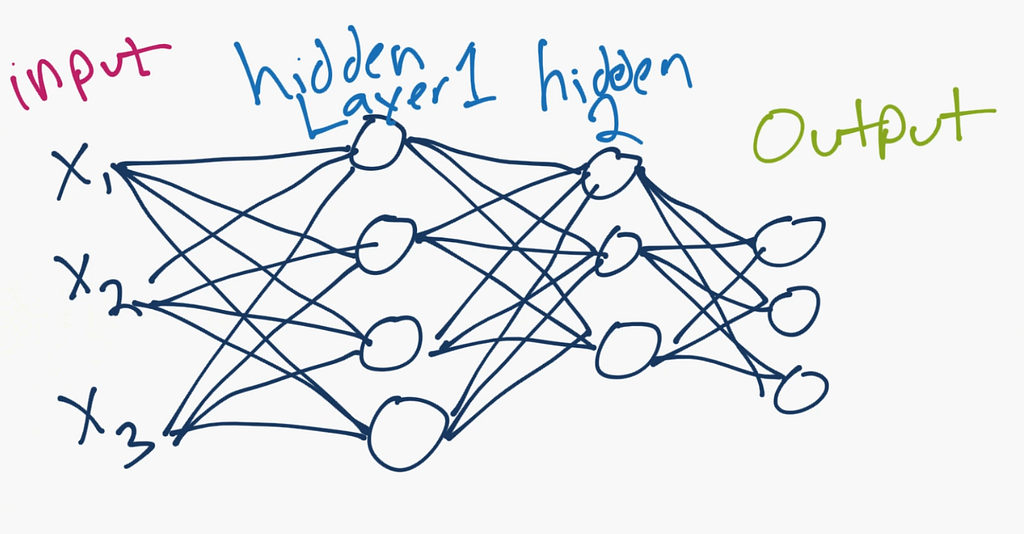Join thousands of students in our LangChain and Vector DBs in Production course, with over 50+ lessons and practical projects for FREE!.

## PublicationMachine Learning

# How to Build and Train Your First Neural Network

Last Updated on January 6, 2023 by Editorial Team

#### Author(s): Davuluri Hemanth Chowdary

Before we dive into the process of building the neural network, there are few prerequisites which you need to know.

Make sure you go through those topics for a better understanding of the concept.

### What is a Neural Network?

Neural networks are a set of algorithms used to recognize patterns in the unstructured data. These neural networks try to mimic the human brain as they are modeled after the human brain.

1. Artificial Neural Networks (ANN)
2. Convolution Neural Networks (CNN)
3. Recurrent Neural Networks (RNN)

1. They can learn from giving train data and find similarities easily.
2. Even if a neuron is not responding, it can still manage to get the output.
3. Parallel processes can run without affecting the overall performance.

#### Applications of Neural Networks:

1. Image processing
2. Speech Recognition
3. pattern prediction
4. Clustering etc.

As a beginner, ANN will be pretty easy and simple to understand. So, I will be concentrating more on ANN.

#### What is an Artificial Neural Network?

These are the simplest forms of neural networks. The information is passed only in one direction with the help of input nodes until it makes it to the output. This type of neural network is also called a Feed-Forward Neutral network.

Let’s take a look at the simple ANN.

ANN consists of three main layers, The Input, Hidden, and Output layer.ANN mostly solves problems related to Image, Text, and Tabular data.

#### Working of ANN

The input layer receives information from outside and goes through two or more hidden layers. These hidden layers transform the input into the output.

Before we dive deeper. Let us see one of the practical usages.

I’m going to use the MNIST sample digits data set to explain ANN’s. We are going to classify images into 10 categories using ANN’s.

#### Step 1: Import all the required libraries

You can find the installation procedure of Keras in the following link.

The MNIST data-set comes preloaded in Keras, in the form of Numpy arrays.

The data-set is split into test and train sets.

Once take a look at the train and test data-set.

#### Step 3: Creating the Neural Network

Layers are the most important building blocks of a neural network. You can think of them as a filter for the data. Take a look at our network. We have a sequence of two dense connected layers, which are fully connected. The last layers are 10-way softmax layer, which gives returns an array of 10 probability scores.

#### What does activation mean?

These are mathematical functions that determine the output of the neural network. These determine the output of a deep learning model, its accuracy, and also the computational efficiency of the model.

1. Relu
2. Sigmoid
3. Softmax
4. Linear

#### Step 4: Network Compilation

The compile function takes three arguments: optimizer, loss, and metrics.

1. Optimizer: These are certain algorithms that are used to change the attributes of the neural network to decrease the loss rate.
2. Loss: This is used to compute the quantity that a model should seek to minimize during training.
3. Metrics: This is used to judge the performance of the model.

#### Step 5: Reshaping the input data

We have to pre-process the data by reshaping and scaling so the values are from 0 to 1.

The original shape of data is (60000, 28, 28) of type unit 8. We have to convert it into (10000, 28 * 28) of float type.

#### Step 6: Preparing the labels

The test and train labels are converted to categorical values.

#### Step 7: Training the model

The fit function takes the training images, training labels, epochs, and batch size as the arguments.

Epochs: The fit function takes the training images, training labels, epochs, and batch size as the arguments.

To check the network accuracy on test data use this line of code.

```test_loss, test_acc = network.evaluate(test_images, test_labels)
print('test_acc:', test_acc)```

The test accuracy is about 98.9 percent.

#### Step 8: Save your model

`network.save("model.h5")`

You can reuse your model after saving it. You need not train the model from the beginning.

#### Step 9: How to load the model

`from keras.models import load_model`
`# load model`
`model = load_model('model.h5')`

After loading the model, its the same process as you did in step 7.

### Conclusion:

I hope you understood the basics and got a bit of clarity on Neural networks. Thanks for reading till the end, and stay tuned for more interesting articles.

How to Build and Train Your First Neural Network was originally published in Towards AI — Multidisciplinary Science Journal on Medium, where people are continuing the conversation by highlighting and responding to this story.

Published via Towards AI